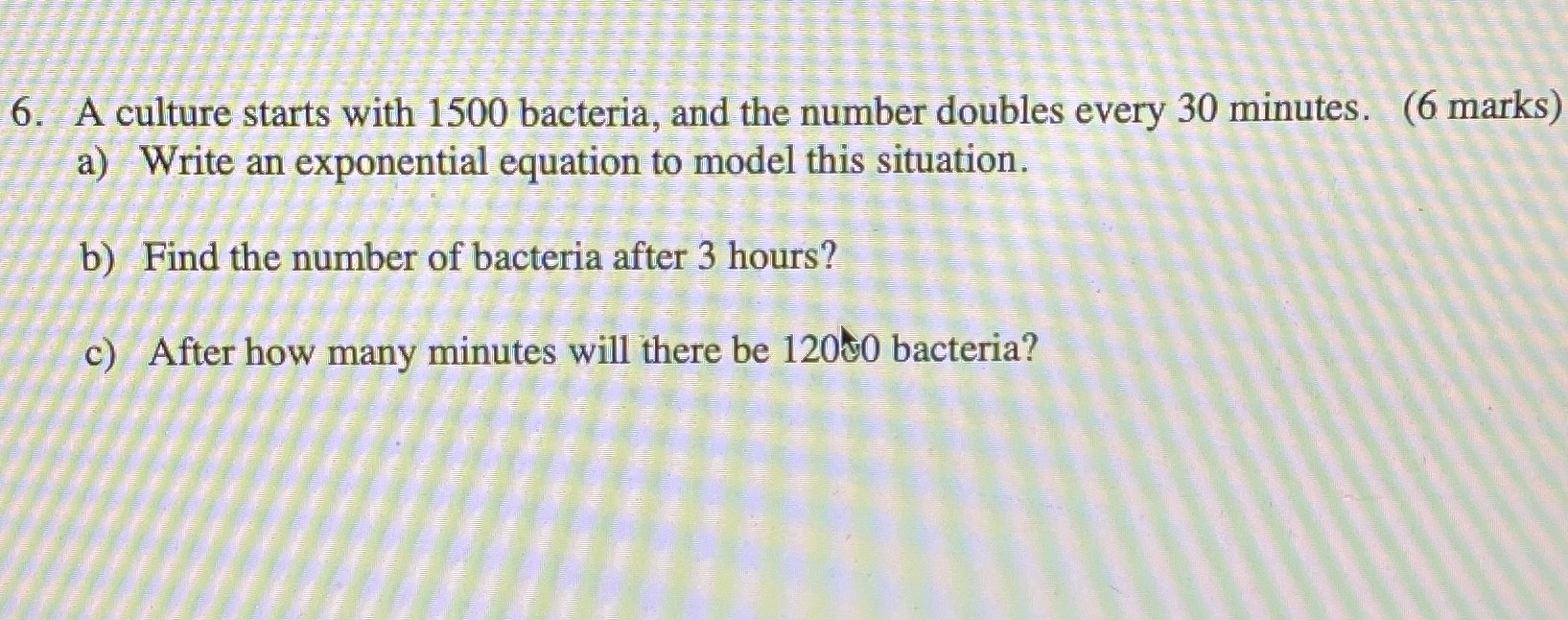### ¿Todavía tienes preguntas de matemáticas?

Pregunte a nuestros tutores expertos
Algebra
PreguntaA culture starts with $$1500$$ bacteria, and the number doubles every $$30$$ minutes.

a) Write an exponential equation to model this situation.

b) Find the number of bacteria after $$3$$ hours?

c) After how many minutes will there be $$120$$ t $$0$$ bacteria?

a) $$1500(2)^{\frac{t}{30} }$$